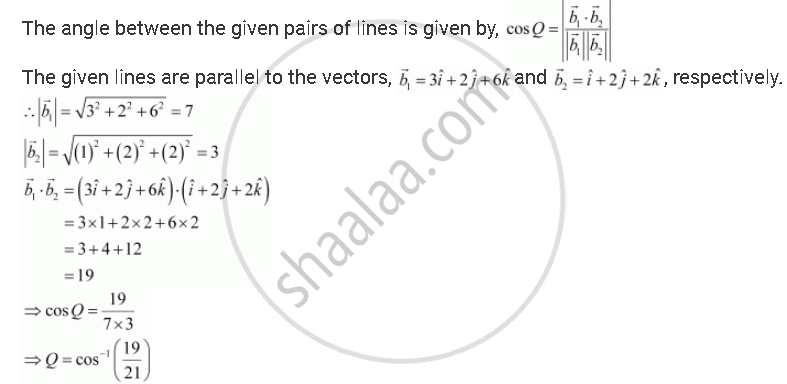Share

# Find the Angle Between the Following Pairs of Lines: Vecr = 2hati - 5hatj + Hatk + Lambda(3hati - 2hatj + 6hatk) and Vecr = 7hati - 6hatk + Mu(Hati + 2hatj + 2hatk) - CBSE (Science) Class 12 - Mathematics

#### Question

Find the angle between the following pairs of lines:

vecr = 2hati - 5hatj + hatk + lambda(3hati - 2hatj + 6hatk) and vecr = 7hati - 6hatk + mu(hati + 2hatj + 2hatk)

#### Solution

Let Q be the angle between the given lines.Is there an error in this question or solution?

#### APPEARS IN

NCERT Solution for Mathematics Textbook for Class 12 (2018 to Current)
Chapter 11: Three Dimensional Geometry
Q: 10.1 | Page no. 478

#### Video TutorialsVIEW ALL 

Solution Find the Angle Between the Following Pairs of Lines: Vecr = 2hati - 5hatj + Hatk + Lambda(3hati - 2hatj + 6hatk) and Vecr = 7hati - 6hatk + Mu(Hati + 2hatj + 2hatk) Concept: Angle Between Two Lines.
S